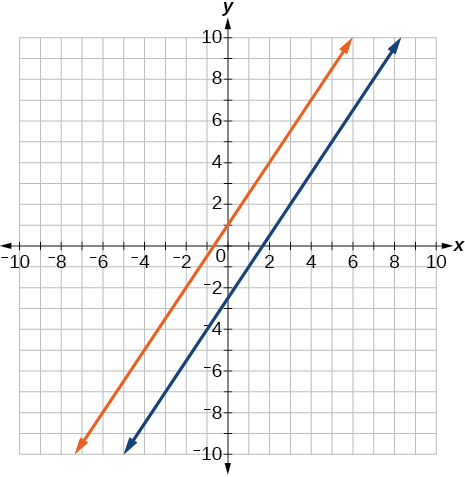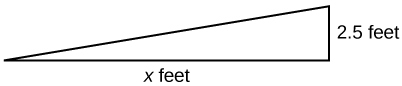# 2.2 Linear equations in one variable  (Page 8/15)

 Page 8 / 15

$\frac{x+2}{4}-\frac{x-1}{3}=2$

$x=-14$

For the following exercises, solve each rational equation for $\text{\hspace{0.17em}}x.\text{\hspace{0.17em}}$ State all x -values that are excluded from the solution set.

$\frac{3}{x}-\frac{1}{3}=\frac{1}{6}$

$2-\frac{3}{x+4}=\frac{x+2}{x+4}$

$x\ne -4;$ $x=-3$

$\frac{3}{x-2}=\frac{1}{x-1}+\frac{7}{\left(x-1\right)\left(x-2\right)}$

$\frac{3x}{x-1}+2=\frac{3}{x-1}$

$x\ne 1;$ when we solve this we get $\text{\hspace{0.17em}}x=1,$ which is excluded, therefore NO solution

$\frac{5}{x+1}+\frac{1}{x-3}=\frac{-6}{{x}^{2}-2x-3}$

$\frac{1}{x}=\frac{1}{5}+\frac{3}{2x}$

$x\ne 0;$ $x=\frac{-5}{2}$

For the following exercises, find the equation of the line using the point-slope formula.

Write all the final equations using the slope-intercept form.

$\left(0,3\right)\text{\hspace{0.17em}}$ with a slope of $\text{\hspace{0.17em}}\frac{2}{3}$

$\left(1,2\right)\text{\hspace{0.17em}}$ with a slope of $\text{\hspace{0.17em}}\frac{-4}{5}$

$y=\frac{-4}{5}x+\frac{14}{5}$

x -intercept is 1, and $\text{\hspace{0.17em}}\left(-2,6\right)$

y -intercept is 2, and $\text{\hspace{0.17em}}\left(4,-1\right)$

$y=\frac{-3}{4}x+2$

$\left(-3,10\right)\text{\hspace{0.17em}}$ and $\text{\hspace{0.17em}}\left(5,-6\right)$

$y=\frac{1}{2}x+\frac{5}{2}$

parallel to $\text{\hspace{0.17em}}y=2x+5\text{\hspace{0.17em}}$ and passes through the point $\text{\hspace{0.17em}}\left(4,3\right)$

perpendicular to $\text{\hspace{0.17em}}\text{3}y=x-4\text{\hspace{0.17em}}$ and passes through the point $\text{\hspace{0.17em}}\left(-2,1\right)$ .

$y=-3x-5$

For the following exercises, find the equation of the line using the given information.

$\left(-2,0\right)\text{\hspace{0.17em}}$ and $\text{\hspace{0.17em}}\left(-2,5\right)$

$\left(1,7\right)\text{\hspace{0.17em}}$ and $\text{\hspace{0.17em}}\left(3,7\right)$

$y=7$

The slope is undefined and it passes through the point $\text{\hspace{0.17em}}\left(2,3\right).$

The slope equals zero and it passes through the point $\text{\hspace{0.17em}}\left(1,-4\right).$

$y=-4$

The slope is $\text{\hspace{0.17em}}\frac{3}{4}\text{\hspace{0.17em}}$ and it passes through the point $\text{\hspace{0.17em}}\text{(1,4)}\text{.}$

$\left(-1,3\right)\text{\hspace{0.17em}}$ and $\text{\hspace{0.17em}}\left(4,-5\right)$

$8x+5y=7$

## Graphical

For the following exercises, graph the pair of equations on the same axes, and state whether they are parallel, perpendicular, or neither.

$\begin{array}{l}\\ \begin{array}{l}y=2x+7\hfill \\ y=\frac{-1}{2}x-4\hfill \end{array}\end{array}$

$\begin{array}{l}3x-2y=5\hfill \\ 6y-9x=6\hfill \end{array}$Parallel

$\begin{array}{l}y=\frac{3x+1}{4}\hfill \\ y=3x+2\hfill \end{array}$

$\begin{array}{l}x=4\\ y=-3\end{array}$Perpendicular

## Numeric

For the following exercises, find the slope of the line that passes through the given points.

$\left(5,4\right)\text{\hspace{0.17em}}$ and $\text{\hspace{0.17em}}\left(7,9\right)$

$\left(-3,2\right)\text{\hspace{0.17em}}$ and $\text{\hspace{0.17em}}\left(4,-7\right)$

$m=\frac{-9}{7}$

$\left(-5,4\right)\text{\hspace{0.17em}}$ and $\text{\hspace{0.17em}}\left(2,4\right)$

$\left(-1,-2\right)\text{\hspace{0.17em}}$ and $\text{\hspace{0.17em}}\left(3,4\right)$

$m=\frac{3}{2}$

$\text{\hspace{0.17em}}\left(3,-2\right)$ and $\text{\hspace{0.17em}}\left(3,-2\right)$

For the following exercises, find the slope of the lines that pass through each pair of points and determine whether the lines are parallel or perpendicular.

${m}_{1}=\frac{-1}{3},\text{ }{m}_{2}=3;\text{ }\text{Perpendicular}\text{.}$

## Technology

For the following exercises, express the equations in slope intercept form (rounding each number to the thousandths place). Enter this into a graphing calculator as Y1, then adjust the ymin and ymax values for your window to include where the y -intercept occurs. State your ymin and ymax values.

$0.537x-2.19y=100$

$y=0.245x-45.662.\text{\hspace{0.17em}}$ Answers may vary.

$4,500x-200y=9,528$

$\frac{200-30y}{x}=70$

$y=-2.333x+6.667.\text{\hspace{0.17em}}$ Answers may vary.

## Extensions

Starting with the point-slope formula $\text{\hspace{0.17em}}y-{y}_{1}=m\left(x-{x}_{1}\right),$ solve this expression for $\text{\hspace{0.17em}}x\text{\hspace{0.17em}}$ in terms of $\text{\hspace{0.17em}}{x}_{1},y,{y}_{1},$ and $\text{\hspace{0.17em}}m.$

Starting with the standard form of an equation solve this expression for y in terms of $\text{\hspace{0.17em}}A,B,C,\text{\hspace{0.17em}}$ and $\text{\hspace{0.17em}}x.\text{\hspace{0.17em}}$ Then put the expression in slope-intercept form.

$y=\frac{-A}{B}x+\frac{C}{B}$

Use the above derived formula to put the following standard equation in slope intercept form: $\text{\hspace{0.17em}}7x-5y=25.$

Given that the following coordinates are the vertices of a rectangle, prove that this truly is a rectangle by showing the slopes of the sides that meet are perpendicular.

$\left(-1,1\right),\left(2,0\right),\left(3,3\right)\text{,}$ and $\text{\hspace{0.17em}}\left(0,4\right)$

Yes they are perpendicular.

Find the slopes of the diagonals in the previous exercise. Are they perpendicular?

## Real-world applications

The slope for a wheelchair ramp for a home has to be $\text{\hspace{0.17em}}\frac{1}{12}.\text{\hspace{0.17em}}$ If the vertical distance from the ground to the door bottom is 2.5 ft, find the distance the ramp has to extend from the home in order to comply with the needed slope.30 ft

If the profit equation for a small business selling $\text{\hspace{0.17em}}x\text{\hspace{0.17em}}$ number of item one and $\text{\hspace{0.17em}}y\text{\hspace{0.17em}}$ number of item two is $\text{\hspace{0.17em}}p=3x+4y,$ find the $\text{\hspace{0.17em}}y\text{\hspace{0.17em}}$ value when

For the following exercises, use this scenario: The cost of renting a car is $45/wk plus$0.25/mi traveled during that week. An equation to represent the cost would be $\text{\hspace{0.17em}}y=45+.25x,$ where $\text{\hspace{0.17em}}x\text{\hspace{0.17em}}$ is the number of miles traveled.

What is your cost if you travel 50 mi?

$57.50 If your cost were $\text{\hspace{0.17em}}\text{}63.75,$ how many miles were you charged for traveling? Suppose you have a maximum of$100 to spend for the car rental. What would be the maximum number of miles you could travel?

220 mi

More example of algebra and trigo
What is Indices
If one side only of a triangle is given is it possible to solve for the unkown two sides?
please I need help in maths
Okey tell me, what's your problem is?
Navin
the least possible degree ?
(1+cosA)(1-cosA)=sin^2A
good
Neha
why I'm sending you solved question
Mirza
Teach me abt the echelon method
Khamis
exact value of cos(π/3-π/4)
What is differentiation?
modul questions trigonometry
(1+cosA)(1-cosA)=sin^2A
BINCY
differentiate f(t)=1/4t to the power 4 +8
I need trigonometry,polynomial
ok
Augustine
Why is 7 on top
simplify cot x / csc x
👉🌹Solve🌻 Given that: cotx/cosx =cosx/sinx/cosx =1/sinx =cosecx Ans.
Vijay
what is the period of cos?
Patrick
simplify: cot x/csc x
Catherine
sorry i didnt realize you were actually asking someone else to put their question on here. i thought this was where i was supposed to.
Catherine
some to dereve formula for bulky density
kurash
Solve Given that: cotx/cosx =cosx/sinx/cosx =1/sinx =cosecx Ans.
Vijay
if tan alpha + beta is equal to sin x + Y then prove that X square + Y square - 2 I got hyperbole 2 Beta + 1 is equal to zero
questions
Thamarai
ok
AjAByByBy